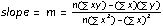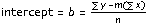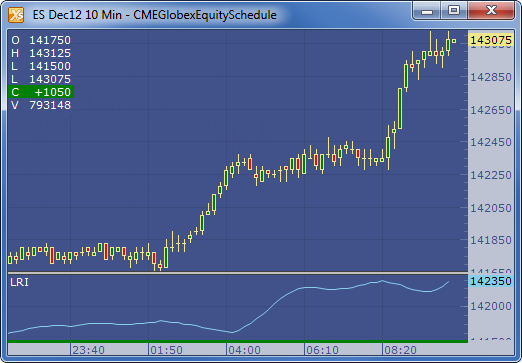← Back to X_TRADER® Help Library

X_STUDY® Documentation

Linear Regression Intercept (LRI)

Description

Linear regression is a statistical tool used to help predict future values from past values. It is commonly used as a quantitative way to determine the underlying trend and when prices are overextended. A linear regression trendline uses the least squares method to plot a straight line through prices so as to minimize the distances between the prices and the resulting trendline. This linear regression intercept indicator plots the intercept for the trendline for each data point.

Formula

The best fit line associated with the n points (x1, y1), (x2, y2), . . . , (xn, yn) has the form y = mx + bExample